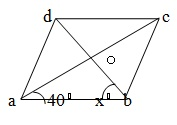# Geometry - Online Quiz

Following quiz provides Multiple Choice Questions (MCQs) related to Geometry. You will have to read all the given answers and click over the correct answer. If you are not sure about the answer then you can check the answer using Show Answer button. You can use Next Quiz button to check new set of questions in the quiz.Q 1 - Two Lines AB and CD intersect at O. If ∠AOC =50⁰,Then ∠ BOD and ∠AOD are respectively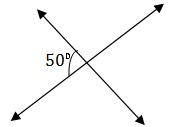A - 130⁰ ,50⁰

B - 50⁰, 130⁰

C - 60⁰, 120⁰

D - 40⁰, 140⁰

### Explanation

```∠ BOD=∠ AOC (vert . opp.  ∠s) =50⁰
∠AOC +∠AOD = 180⁰ ⇒ 50⁰ +∠AOD= 180⁰ ⇒∠AOD=130⁰
∴ ∠ BOD= 50⁰ and ∠AOD = 130⁰.```

Q 2 - In the given figure ,what is the value of x, if 4x= 5y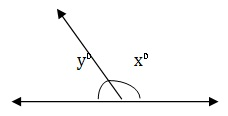A - 100⁰

B - 105⁰

C - 110⁰

D - 115⁰

### Explanation

`x+y = 180 ⇒ x+ 4/5 x = 180 ⇒ 5x +4x = 900 ⇒ 9x=900 ⇒ x = 100.`

Q 3 - In the given figure , AB || CD, ∠ABE =35⁰, ∠CDE = 65⁰ and ∠BED =x⁰. Then, x= ?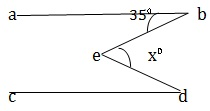A - 30⁰

B - 100⁰

C - 125⁰

D - 145⁰

### Explanation

```Draw GEH ||AB||CD.
∠ BHE =∠ ABE = 35⁰ (alt .∠s)
∠ DEH =∠ CDE = 65⁰ (alt .∠s)
∴∠x=∠ BEH + ∠DEH = (35⁰ +65⁰)=100⁰.```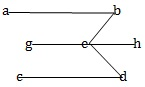Q 4 - The angles of a triangle are in the ratio 2:3:7. The measure OF the smallest angle is:

A - 90⁰

B - 60⁰

C - 45⁰

D - 30⁰

### Explanation

```let the angles be (2x)⁰,(3x)⁰ and (7x)⁰. Then,
2x+3x+7x =180 ⇒ 12x =180 ⇒ x=15
Smallest angle = (2x)⁰ = 30⁰```

Q 5 - The angle of a triangle are 3x⁰, (2x-7)⁰ and (4x-11)⁰. The value of x is :

A - 18⁰

B - 20⁰

C - 22⁰

D - 30⁰

### Explanation

```The sum of the angle of a triangle is 180⁰.
∴ 3x = 2x - 7 + 4x -11 = 180 ⇒ 9x =162 ⇒ x = 18.
Hence, x = 18.```

Q 6 - Two poles of heights 6m and 11m stand vertically on a plane ground. If the distance between their feet is 12m , what is the distance between their tops?

A - 13 m

B - 14 m

C - 15 m

D - 12.8 m

### Explanation

```Let AB and CD be the poles such that
AB = 6m , CD = 11 m and BD =12m
Draw AE ⊥ CD . Then , AE = BD = 12m
CE = CD - DE = CD - AB = (11 - 6) m =5m.
from right   AEC we have
AC2 = AE2  + CE2 = (12)2 + 52 = (114 +25)=169
⇒ Ac =  √169 = 13m
∴ Distance between their tops= 13m```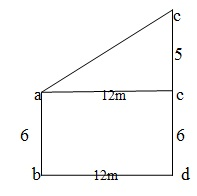Q 7 - A chord of length 30cm is at a distance of 8cm from the center of a circle . The radius of the circle is

A - 11 cm

B - 13 cm

C - 15 cm

D - 17 cm

### Explanation

```Let O be the centre of the circle and AB  be the chord. Draw OL ⊥  AB.
Then AL= 1/2 AB = (1/2 *30)cm =15 cm  and OL = 8cm.
OA2 = OL2 +AL2= 82 + (15)2  = (64  + 225 ) =289
⇒ OA = √289  = 17cm.
∴ Radius of the circle is 17 cm.```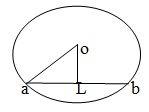Q 8 - In the given figure ,ABCD is a cyclic quadrilateral in which AB || DC and ∠ BAD = 100⁰. Then , ∠ ABC=?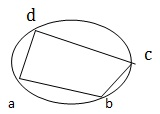A - 80⁰

B - 100⁰

C - 75⁰

D - 150⁰

### Explanation

```AB   DC and AD  is the transversal.
Opposite angles of a cyclic quadrilateral are supplementary.
∴ ∠ADC +∠ABC = 180⁰ ⇒ 80⁰+ ∠ABC =180⁰ ⇒ ABC = 100⁰.```

Q 9 - In the given figure, chords AB and CD of a circle intersect externally at P. If AB =6cm, CD = 3cm and PD= 5cm then PB= ?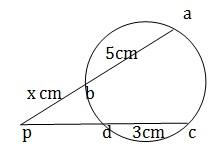A - 5 cm

B - 6.25 cm

C - 6 cm

D - 4 cm

### Explanation

```PA * PB + PC *PD ⇒ (x+6 ) * x=8* 5 ⇒ x2 +6x - 40 =0
⇒ (x+10) (x-4) =0 ⇒ x=4
∴ PB= 4 cm```

Q 10 - In The adjoining figure, ABCD is a rhombus whose diagonals intersect at O. IF ∠OAB =40⁰ and ∠ABO =x⁰, then X= ?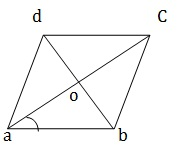A - 50⁰

B - 35⁰

C - 40⁰

D - 45⁰

```We know that the diagonals of a  rhombus